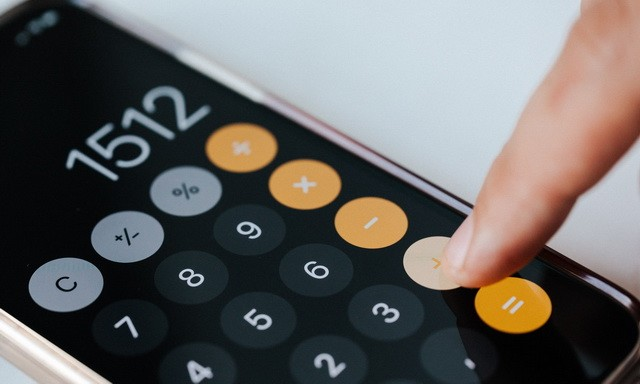Calculator is one of the basic yet very important apps which we use for calculations. The default calculator app in Android smartphones is good enough but if you are looking for advanced calculations then you will need to go for third-party calculator apps. There are hundreds of calculator apps available on Google Play Store and we have handpicked the best ones for you. In this comprehensive guide, we will share the best calculator apps for Android.

## MyScript Calculator 2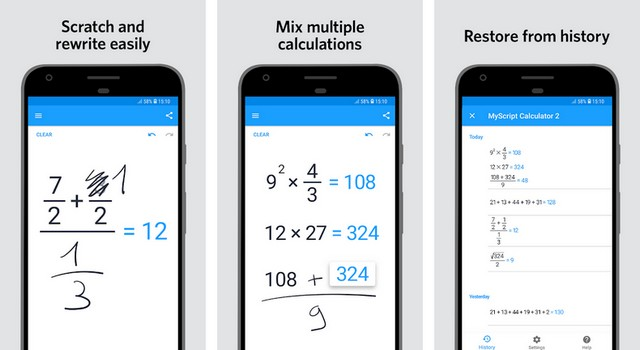MyScript Calculator 2 is one of the best calculator apps for Android. The best thing about this calculator is that it turns the display of your smartphone into an interactive piece of paper where you can do various calculations. You can easily write the calculations with the keyboard and you can erase them with scratch-out gestures. The app also automatically saves the results into memory so you can reuse them anytime you want.

## HiPER Scientific Calculator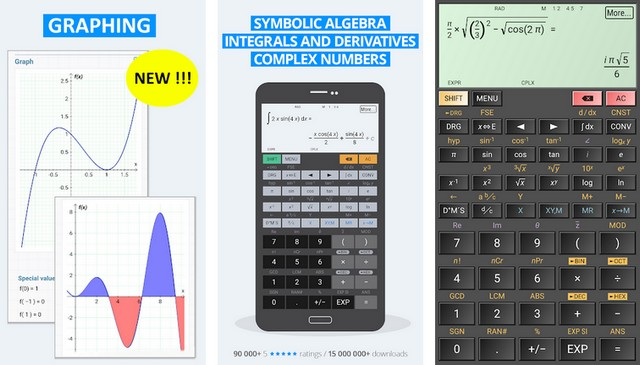HiPER Scientific Calculator is one of the best scientific calculator apps for Android. It is basically the scientific calculator on your device and its layout is also very similar to the scientific calculator. HiPER Calculator comes with tons of functions such as mixed numbers, operator priority, derivatives and integrals, complex numbers, trigonometric and hyperbolic functions, powers, roots, logical operation, bitwise shifts and rotations, complex numbers and many more. If you are looking for a good scientific calculator then you can’t go wrong with HiPER Scientific Calculator.

## All-In-One Calculator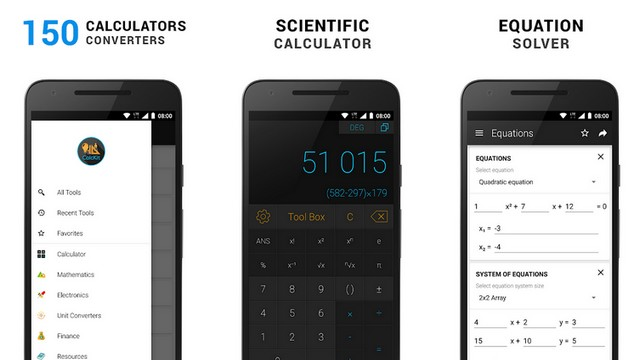All-In-One Calculator is one of the best calculator apps for Android. As the name suggests, it is an all-in-one solution for all your calculator needs. It comes with a massive 150 calculators and converters which you can use to calculate almost everything. The app features the calculators for Geometry, Algebra, Equations, Electronics, Finance, and many more. It also features the fair amount of current converters that support more than 160 currencies. You can use the app for free but it comes with ads and in-app purchases.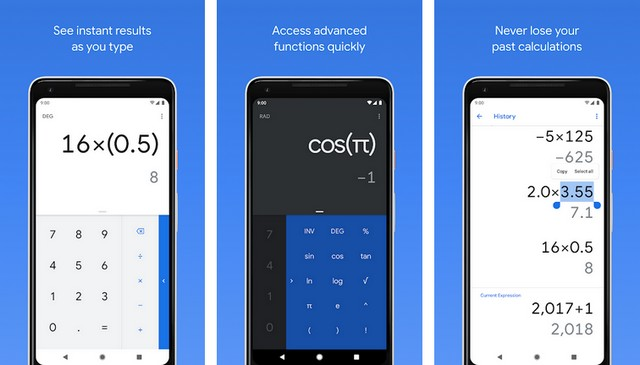Google Calculator is one of the simplest calculator apps available on Google Play. It is not an advanced app and it can do basic operations such as addition, subtraction, multiplication, and division. The app comes with a very minimalistic User Interface which makes it easier to operate the calculator. It also comes with the history of the operations so that you can use them during the calculations. Google Calculator is also completely free to use.

## Photomath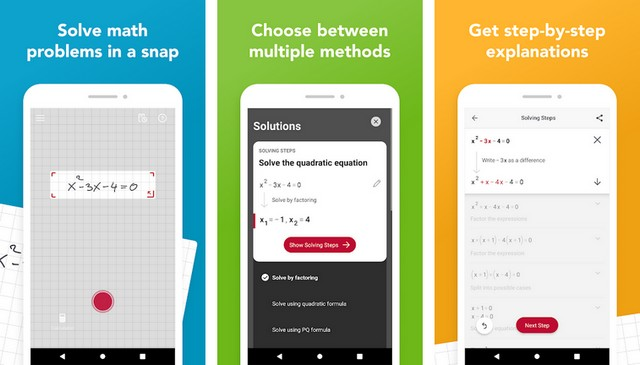Photomath is one of the best calculator apps for Android. The best thing about this app is that it is not just an ordinary calculator app but it is also a very comprehensive math problem-solving app that you can use to find answers for your work exercises. It provides a step-by-step explanation for every solution which makes it ideal for math students. Photomath is free to use but it comes with in-app purchases.

## Calculator++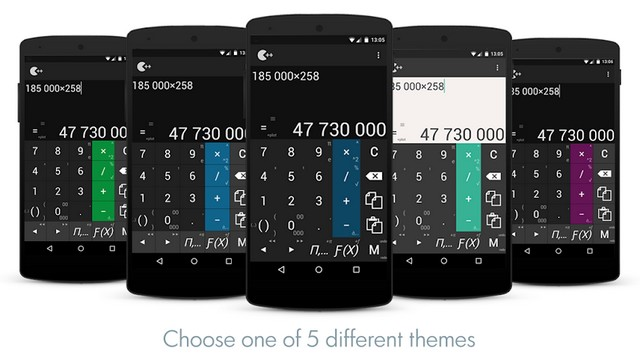Calculator++ is one of the better calculator apps which you can use for calculations. The best thing about this app is that it comes with the two layouts: Standard and Engineer Layout. You can switch between both layouts easily and pick the one which suits you. It comes with support for basic math calculation, variables, constants, integrations and differentiate, fractions, simplifying expressions, and complex numbers.

## Calc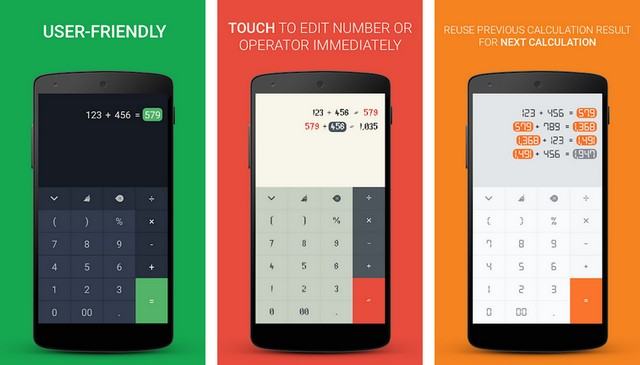Calc is another very popular calculator app for Android. It is a very simple calculator app and it comes with the Material Design theme which looks very attractive. The calculator itself can help you with some basic calculations such as addition, subtraction, multiplication, and division. It also keeps the history of the calculations and also allows you to store it in the note’s app. If you are looking for a simple calculator app then Calc is one the better options.

## ClevClac Calculator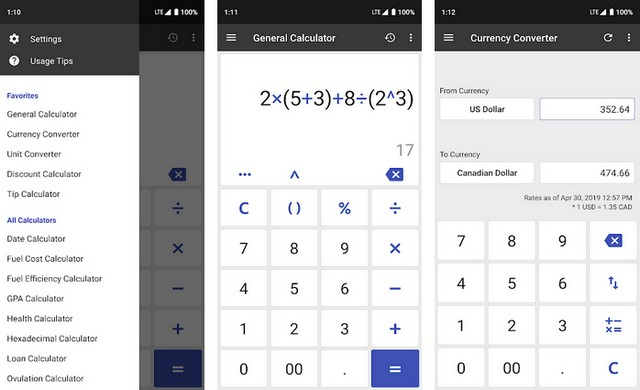ClevClac is one of the best calculator apps for Android. It is a very comprehensive app and it comes with a variety of calculators and converters which can be very useful. The app comes with a fair variety of calculators such as General Calculator, Tip Calculator, Date Calculator, Fuel Cost Calculator, GPA Calculator, Hexadecimal Calculator, Loan Calculator, and more. It also comes with the simple and intuitive User Interface which makes it easier to use. If you are looking for a comprehensive calculator app then you can’t go wrong with ClevClac.

## Desmos Graphing Calculator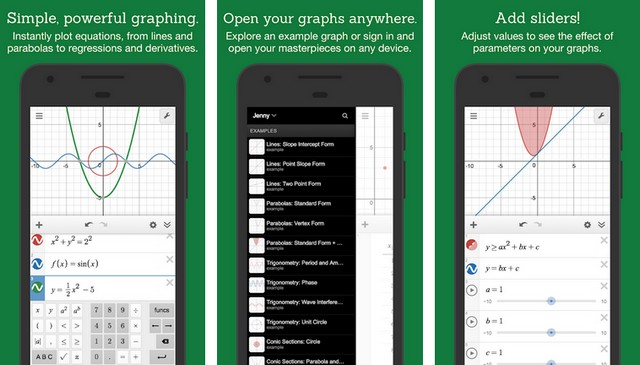Desmos Graphing Calculator is one of the best graphing calculators for Android. It is an advanced graphing calculator that features all the features which you can expect from a good graphing calculator. It allows you to plot a variety of graphs such as polar, Cartesian, or parametric graphs. The app also supports the sliders & tables to adjust the value or plot the data. It also features the scientific calculator where you can get the answer by simply typing the equation. Desmos Graphing Calculator is also completely free to use.

## Financial Calculators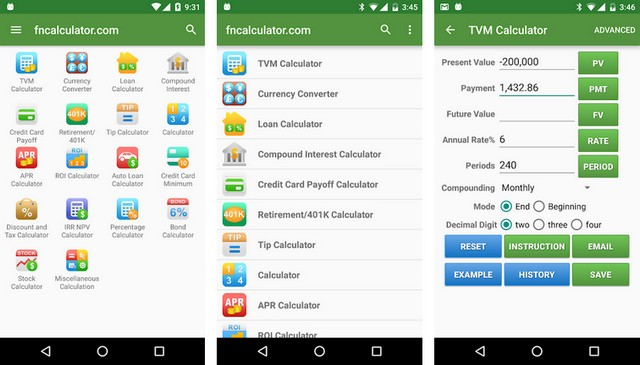Financial Calculators is an all-in-one calculator app that comes with a large collection of calculators. In this app, you will find the calculators such as TVM Calculator, Currency Calculator, Loan Calculator, Compound Interest Calculator, APR Calculator, ROI Calculator, Bond Calculator, Percentage Calculator, Stock Calculator, Retirement Calculator, and many more. The app also comes with a currency converter that allows you to convert any currency you want. You can use the app for free but it comes with ads.